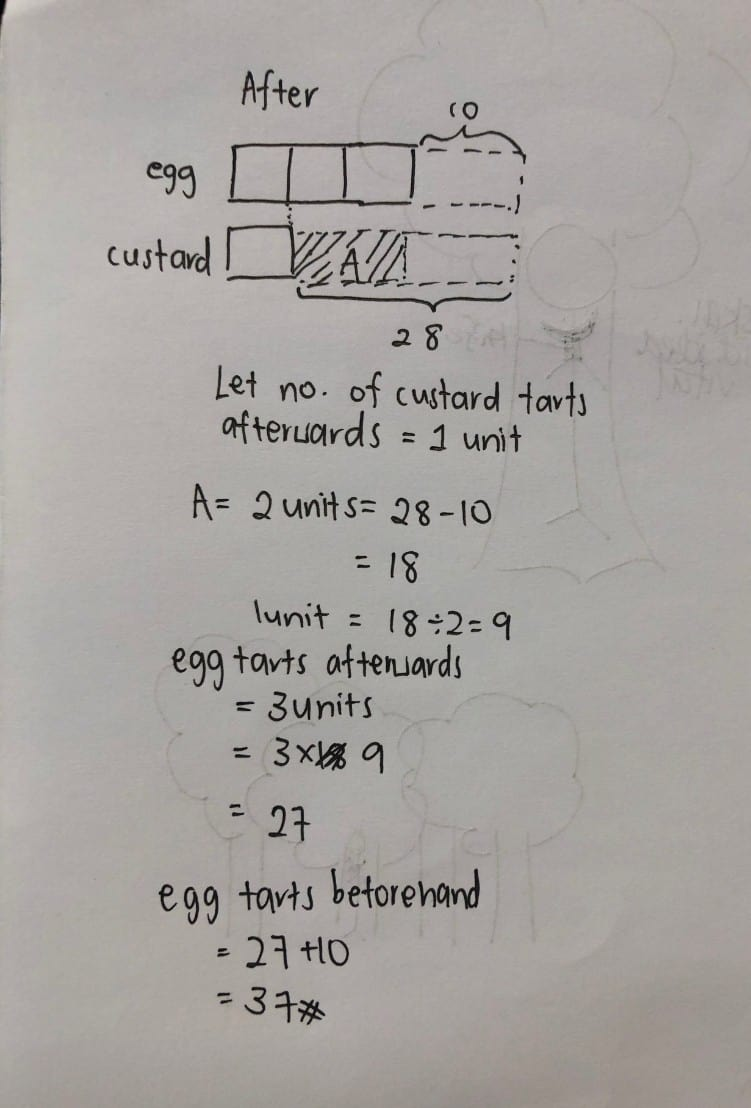# Answer

Let the number of custard tarts (after) = 1u

3u + 10 = 1u + 28

2u = 18

u = 18/2 = 9

Number of egg tarts initially

= 3u + 10

= (3 x 9) + 10

= 27 + 10 = 37 (ans)

0 Replies 0 Likes ✔Accepted Answer

E          C

?           ?                          (at first, same)

-10     -28

3u     :     1u                     (in the end)

3u + 10 = 1u + 28         (work backwards from results)

2u + 10 =         28         (remove 1u from each side for equation to stay balance)

2u          =          18         (remove 10 from each side for equation to stay balance)

1u = 9

3u = 27                           (in the end, egg tarts)

27 + 10 = 37                  (ANSWER 🙂

0 Replies 0 Likes

28 – 10 = 18 (difference in number of tarts given to her neighbour)
3 – 1 = 2 (difference in units)
18/2 = 9 (1 unit of tarts)
9 x 3 + 10 = 37

Ans : 37 egg tarts.

0 Replies 0 LikesHope this helps!

0 Replies 0 Likes